Equations

Forming and solving equations in one unknown

Remember that to solve an equation, you must find a number that can replace the unknown in the equation and make it a true statement.

Equations may be likened to the balance scale,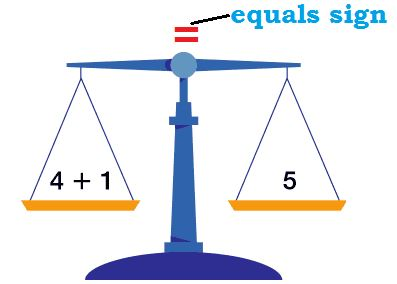The two sides must balance, therefore, whatever you do to one side of an equation, you must do to the other side.

Equation equality rule

Similarly the same quantity or number must be subtracted from both sides of the equation without affecting the equality of the equation.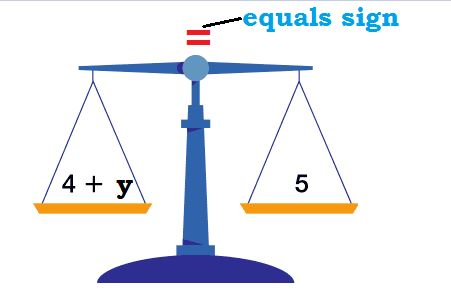The same quantity or number must be added to both sides of the equation without affecting the equality of the equation.

Also divide or multiply both sides of the equation by the same quantity or number (except zero) without affecting the equality of the equation.

Opposite operation rule

If an equation indicates an operation such as addition, subtraction, multiplication, or division, solve for the unknown by using the opposite operation.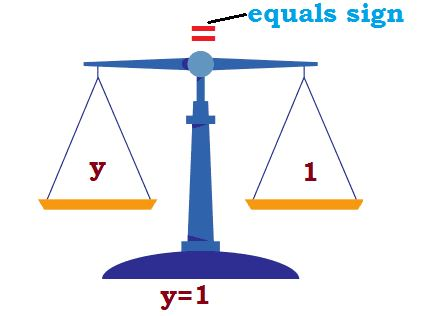For example, if the equation operation is addition, solve for the unknown by using subtraction.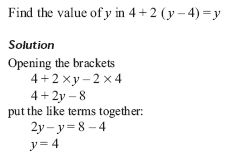Solving equations

Remember the equation equality rule and the  opposite operation rule while solving the equation below.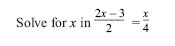And here is the solution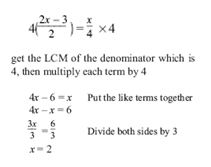Sources

• algebra_solving_equations_balance_scale_2 by Unknown used under Creative Commons Attribution-NonCommercial-NoDerivatives 4.0 International License
• algebra_solving_equations_balance_scale_3 by Unknown used under Creative Commons Attribution-NonCommercial-NoDerivatives 4.0 International License
• algebra_solving_equations_balance_scale_4 by Unknown used under Creative Commons Attribution-NonCommercial-NoDerivatives 4.0 International License
• algebra_solving_equations_balance_scale by Unknown used under Creative Commons Attribution-NonCommercial-NoDerivatives 4.0 International License
• equation_1 by Unknown used under Creative Commons Attribution-NonCommercial-NoDerivatives 4.0 International License
• equation_2 by Unknown used under Creative Commons Attribution-NonCommercial-NoDerivatives 4.0 International License
• equation3 by Unknown used under Creative Commons Attribution-NonCommercial-NoDerivatives 4.0 International License

•